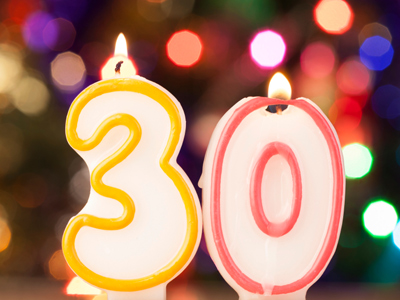30 is the 5th multiple of the number 6.

# Level 3-4 Algebra - Multiples

Factors are an important part of KS3 Maths but so are multiples. So what's the difference. Well, factors and multiples are similar yet opposite. Whereas factors are numbers which another number can be divided into, multiples are the numbers you get after multiplying a number.

If a number appears in a times table, then the number of the times table is a factor of that number. Conversely, all of the numbers in a times table are multiples of that number. Here's an example. The number 8 appears in the 1, 2, 4 and 8 times tables and so 1, 2, 4 and 8 are factors of 8. It follows that 8 is a multiple of 1, 2, 4, and 8. Easy eh?

In the field of algebra, you may well be asked to find 'common multiples'. These are numbers which are multiples of two or more numbers. Things can get a little more complicated but plenty of practise will make you an expert on multiples.

Have a go at this quiz and you will soon understand. Good luck!

1.
Which of the following is a common factor of 15, 30, 45 and 60?
2
3
4
10
5 and 3 can be divided into all the numbers given and the result is always a whole number. Remember that multiples are always whole numbers
2.
Which of the following are common factors of 6, 12, 18 and 24?
2 and 3
3 and 4
4 and 5
5 and 6
Both 2 and 3 will divide into all the numbers given (6, 12, 18 and 24)
3.
The 2nd and 3rd multiples of a number are 22 and 33. What is the number?
5
7
9
11
Simply divide 22 by 2 and 33 by 3. Easy eh?
4.
Which of these numbers is not a multiple of 3 and 4?
12
36
48
50
3 and 4 cannot be divided into 50 to give answers of whole numbers
5.
What are the first 4 multiples of 7?
0, 7, 14, 21
1, 7, 14, 21
7, 14, 21, 28
14, 21, 28, 35
1 x 7 = 7; 2 x 7 = 14; 3 x 7 = 21; 4 x 7 = 28
6.
The first 3 multiples of a number are 8, 16, 24. What is the number?
8
16
24
32
8, 16 and 24 are all in the 8 times table
7.
The 4th and 5th multiples of a number are 24 and 30. What is the number?
3
6
9
10
If you know your times tables then multiples aren't very difficult
8.
Is the number 43 a multiple of the number 11?
Yes
No
Seldom
Often
44 is a multiple of 11 but 43 most definitely is not!
9.
Is 66 a multiple of 3 and 11?
Yes
No
Seldom
Often
Both 3 and 11 will divide into 66 and give results of a whole number
10.
What are the first 3 multiples of 15?
3, 5, 7.5
5, 10, 15
15, 30, 45
30, 60, 90
1 x 15 = 15; 2 x 15 = 30; 3 x 15 = 45
You can find more about this topic by visiting BBC Bitesize - Prime numbers, factors and multiples

Author:  Frank Evans